# Pentagrams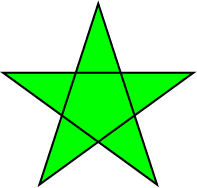A pentagram (also known as a pentangle or a star pentagon) is a geometric shape that looks like a 5 pointed star. It is drawn using five straight line segments.

The pentagram was originally used as a symbol in ancient Greece and Babylon. The early Christians adopted the pentagram symbol, and it has also been used in Taoism and Islam, as shown on the Moroccan flag. Many people think of it as a symbol of witchcraft, but this is not entirely true.

The pentagram is one of the star polygons.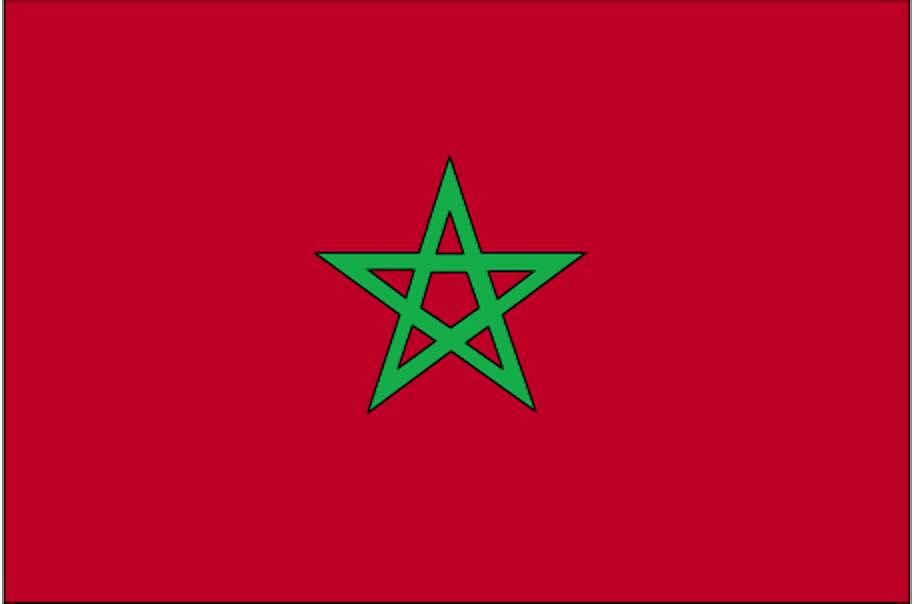## Interesting Geometric Properties of the Pentagram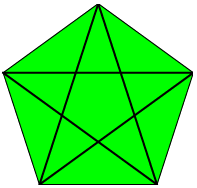You can draw a pentagram by extending the edges of a pentagon until they intersect externally. You can also draw a pentagram inside a pentagon by joining up the vertices internally as shown in the picture on the left.

The picture on the right shows that each pentagram contains a pentagon (not necessarily blue). So, this means that you could (theoretically) draw an infinite sequence of pentagrams, nested, one inside the other, and you could extend it outwards as well. Pretty amazing, isn't it? This might be the reason for the pentagram's supposedly mystical properties.## The Golden Ratio and the Pentagram

The Golden Ratio is a number that has fascinated mathematicians, artists and architects for centuries. The golden ratio is believed to provide the key to producing aesthetically pleasing art. It has been asserted that Leonardo da Vinci used the proportions dictated by the golden ratio when painting the Mona Lisa.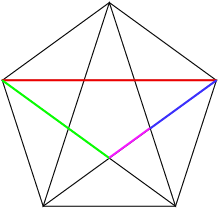The ratios between the various coloured side lengths shown on this pentagram is supposed to equal the golden ratio ($1.618\dots$). I measured the coloured side lengths of this pentagram to be $\text{red}=76$ mm, $\text{green}= 46.5$ mm, $\text{blue} = 29$ mm and $\text{pink} = 18$ mm. I then calculated the ratios. The values I obtained were quite close to the golden ratio. Any differences are probably due to measurement and/or errors in drawing. Here are my ratios:

• $\dfrac{\text{red}}{\text{green}} = 1.634$
• $\dfrac{\text{green}}{\text{blue}} = 1.603$
• $\dfrac{\text{blue}}{\text{pink}} = 1.611$

Why don't you see if you can do better than I did? Draw yourself a regular pentagram, measure these distances, and calculate the ratios.

### Regular and Irregular Pentagrams

Regular pentagrams are pentagrams in which all the sides and angles (in the points) are equal. So far, we've only talked about regular pentagrams, but irregular pentagrams do exist. In fact, they are much easier to draw than regular pentagrams! I drew the irregular pentagram in about 5 seconds. The regular one took me quite a bit longer.

Here's an example: the picture shows regular and irregular pentagrams side by side.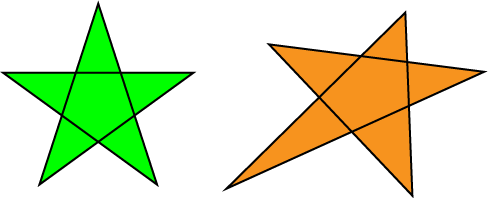### Description

In these chapters you will learn about plane geometry topics such as

• Area (Irregular polygons, plane shapes etc)
• Perimeter
• Conic sections (Circle, Ellipse, Hyperbola etc)
• Polygons (Congruent, polygons, similar, triangles etc)
• Transformations and symmetry (Reflection, symmetry, transformations etc)

etc

Even though these chapters are marked for Year 10 or higher students, several topics are for students in Year 8 or higher

### Audience

Year 10 or higher, suitable for Year 8 + students as well.

### Learning Objectives

You must be logged in as Student to ask a Question.